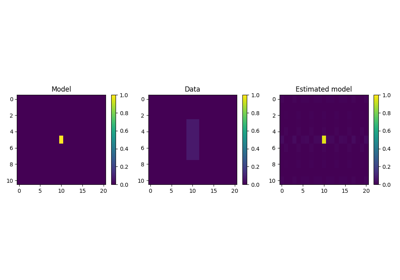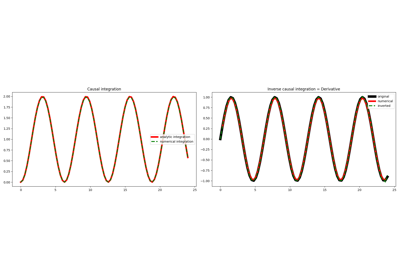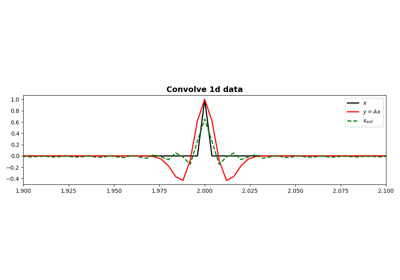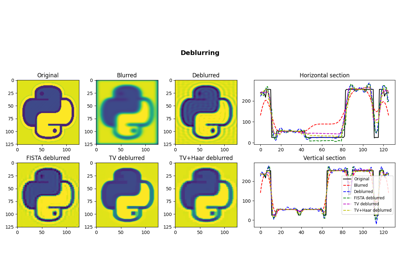# pylops.signalprocessing.ConvolveND¶

class pylops.signalprocessing.ConvolveND(*args, **kwargs)[source]

ND convolution operator.

Apply n-dimensional convolution with a compact filter to model (and data) along the axes of a n-dimensional array.

Parameters
dims

Number of samples for each dimension

hnumpy.ndarray

nd compact filter to be convolved to input signal

offsettuple, optional

Indices of the center of the compact filter

axesint, optional

New in version 2.0.0.

Axes along which convolution is applied

methodstr, optional

Method used to calculate the convolution (direct or fft).

dtypestr, optional

Type of elements in input array.

namestr, optional

New in version 2.0.0.

Name of operator (to be used by pylops.utils.describe.describe)

Notes

The ConvolveND operator applies n-dimensional convolution between the input signal $$d(x_1, x_2, ..., x_N)$$ and a compact filter kernel $$h(x_1, x_2, ..., x_N)$$ in forward model. This is a straighforward extension to multiple dimensions of pylops.signalprocessing.Convolve2D operator.

Attributes
shapetuple

Operator shape

explicitbool

Operator contains a matrix that can be solved explicitly (True) or not (False)

Methods

 __init__(dims, h[, offset, axes, method, ...]) Initialize this LinearOperator. adjoint() Hermitian adjoint. apply_columns(cols) Apply subset of columns of operator cond([uselobpcg]) Condition number of linear operator. conj() Complex conjugate operator div(y[, niter, densesolver]) Solve the linear problem $$\mathbf{y}=\mathbf{A}\mathbf{x}$$. dot(x) Matrix-matrix or matrix-vector multiplication. eigs([neigs, symmetric, niter, uselobpcg]) Most significant eigenvalues of linear operator. matmat(X) Matrix-matrix multiplication. matvec(x) Matrix-vector multiplication. reset_count() Reset counters rmatmat(X) Matrix-matrix multiplication. rmatvec(x) Adjoint matrix-vector multiplication. todense([backend]) Return dense matrix. toimag([forw, adj]) Imag operator toreal([forw, adj]) Real operator tosparse() Return sparse matrix. trace([neval, method, backend]) Trace of linear operator. transpose() Transpose this linear operator.

## Examples using pylops.signalprocessing.ConvolveND¶2D Smoothing

2D SmoothingCausal Integration

Causal IntegrationConvolution

Convolution05. Image deblurring

05. Image deblurring